# RD Sharma solutions for Mathematics for Class 9 chapter 17 - Heron’s Formula [Latest edition]

#### Chapters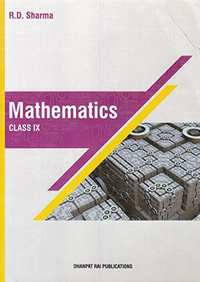## Chapter 17: Heron’s Formula

Exercise 17.1Exercise 17.2Exercise 17.3Exercise 17.4
Exercise 17.1 [Page 8]

### RD Sharma solutions for Mathematics for Class 9 Chapter 17 Heron’s Formula Exercise 17.1 [Page 8]

Exercise 17.1 | Q 1 | Page 8

Find the area of a triangle whose sides are respectively 150 cm, 120 cm and 200 cm ?

Exercise 17.1 | Q 2 | Page 8

Find the area of a triangle whose sides are 9 cm, 12 cm and 15 cm ?

Exercise 17.1 | Q 3 | Page 8

Find the area of a triangle two sides of which are 18 cm and 10 cm and the perimeter is 42cm ?

Exercise 17.1 | Q 4 | Page 8

In a ΔABC, AB = 15 cm, BC = 13 cm and AC = 14 cm. Find the area of ΔABC and hence its altitude on AC ?

Exercise 17.1 | Q 5 | Page 8

The perimeter of a triangular field is 540 m and its sides are in the ratio 25 : 17 : 12. Find the area of the triangle ?

Exercise 17.1 | Q 6 | Page 8

The perimeter of a triangle is 300 m. If its sides are in the ratio 3 : 5 : 7. Find the area of the triangle ?

Exercise 17.1 | Q 7 | Page 8

The perimeter of a triangular field is 240 dm. If two of its sides are 78 dm and 50 dm, find the length of the perpendicular on the side of length 50 dm from the opposite vertex.

Exercise 17.1 | Q 8 | Page 8

A triangle has sides 35 cm, 54 cm and 61 cm long. Find its area. Also, find the smallest of its altitudes ?

Exercise 17.1 | Q 9 | Page 8

The lengths of the sides of a triangle are in the ratio 3 : 4 : 5 and its perimeter is 144 cm. Find the area of the triangle and the height corresponding to the longest side.

Exercise 17.1 | Q 10 | Page 8

The perimeter of an isosceles triangle is 42 cm and its baše is (32) times each of the equal sides. Find the length of each side of the triangle, area of the triangle and the height of the triangle.

Exercise 17.1 | Q 11 | Page 8

Find the area of the shaded region in the given figure.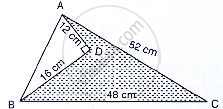Exercise 17.2 [Pages 19 - 20]

### RD Sharma solutions for Mathematics for Class 9 Chapter 17 Heron’s Formula Exercise 17.2 [Pages 19 - 20]

Exercise 17.2 | Q 1 | Page 19

Find the area of a quadrilateral ABCD is which AB = 3 cm, BC = 4 cm, CD = 4 cm, DA = 5 cm and AC = 5 cm.

Exercise 17.2 | Q 2 | Page 19

Area of PQRS = Area of PQR + Area of ΔPQS = (6+9.166)𝑐𝑚2=15.166𝑐𝑚2

Exercise 17.2 | Q 3 | Page 19

The sides of a quadrilateral, taken in order are 5, 12, 14 and 15 meters respectively, and the angle contained by the first two sides is a right angle. Find its are

Exercise 17.2 | Q 4 | Page 19

A park, in the shape of a quadrilateral ABCD, has ∠C = 900, AB = 9 m, BC = 12 m, CD = 5 m and AD = 8 m How much area does it occupy?

Exercise 17.2 | Q 5 | Page 19

Two parallel side of a trapezium are 60 cm and 77 cm and other sides are 25 cm and 26 cm. Find the area of the trapezium.

Exercise 17.2 | Q 6 | Page 19

A rhombus sheet, whose perimeter is 32 m and whose one diagonal is 10 m long, is painted on both sides at the rate of Rs 5 per m2. Find the cost of painting.

Exercise 17.2 | Q 7 | Page 20

Find the area of a quadrilateral ABCD in which AD = 24 cm, ∠BAD = 90° and BCD forms an equilateral triangle whose each side is equal to 26 cm. (Take √3 = 1.73)

Exercise 17.2 | Q 8 | Page 20

Find the area of a quadrilateral ABCD in which AB = 42 cm, BC = 21 cm, CD = 29 cm, DA =34 cm and diagonal BD =20 cm.

Exercise 17.2 | Q 9 | Page 20

The adjacent sides of a parallelogram ABCD measure 34 cm and 20 cm, and the diagonal AC measures 42 cm. Find the area of the parallelogram.

Exercise 17.2 | Q 10 | Page 20

Find the area of the blades of thc magnetic compass shown in Fig.. 12.27. (Take √11 = 3.32).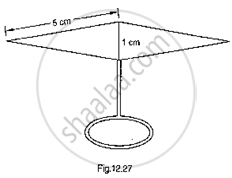Exercise 17.2 | Q 11 | Page 20

A triangle and a parallelogram have the same base and the same area. If the sides of the triangle are 13 cm, 14 cm and 15 cm and the parallelogram stands on the base 14 cm, find the height of the parallelogram.

Exercise 17.2 | Q 12 | Page 20

Find the area of a rhombus whose perimeter is 80 m and one of whose diagonal is 24 m.

Exercise 17.2 | Q 13 | Page 20

Find the perimeter and area of the quadrilateral ABCD in which AB = 17 cm, AD =9 cm, CD = l2cm, ∠ACB = 90° and AC=l5cm.

Exercise 17.2 | Q 14 | Page 20

A hand fan is made by stitching lo equal size triangular strips of two different types of paper as shown in Fig. 12.28. The dimensions of equal strips are 25 cm, 25 cm and 14 cm. Find the area of each type of paper needed to make the hand fan.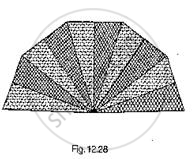Exercise 17.3 [Page 24]

### RD Sharma solutions for Mathematics for Class 9 Chapter 17 Heron’s Formula Exercise 17.3 [Page 24]

Exercise 17.3 | Q 1 | Page 24

Find the area of a triangle whose base and altitude are 5 cm and 4 cm respectively.

Exercise 17.3 | Q 2 | Page 24

Find the area of a triangle whose sides are 3 cm, 4 cm and 5 cm respectively.

Exercise 17.3 | Q 3 | Page 24

Find the area of an isosceles triangle having the base x cm and one side y cm.

Exercise 17.3 | Q 4 | Page 24

Find the area of an equilateral triangle having each side 4 cm.

Exercise 17.3 | Q 5 | Page 24

Find the area of an equilateral triangle having each side x cm.

Exercise 17.3 | Q 6 | Page 24

The perimeter of a triangullar field is 144 m and the ratio of the sides is 3 : 4 : 5. Find the area of the field.

Exercise 17.3 | Q 7 | Page 24

Find the area of an equilateral triangle having altitude h cm.

Exercise 17.3 | Q 8 | Page 24

Let Δ be the area of a triangle. Find the area of a triangle whose each side is twice the side of the given triangle.

Exercise 17.3 | Q 9 | Page 24

If each side of a triangle is doubled, the find percentage increase in its area.

Exercise 17.3 | Q 10 | Page 24

If each side of a equilateral triangle is tripled then what is the percentage increase in the area of the triangle?

Exercise 17.4 [Pages 24 - 25]

### RD Sharma solutions for Mathematics for Class 9 Chapter 17 Heron’s Formula Exercise 17.4 [Pages 24 - 25]

Exercise 17.4 | Q 1 | Page 24

Mark the correct alternative in each of the following:
The sides of a triangle are 16 cm, 30 cm, 34 cm. Its area is

• 225 cm2

• 225  $\sqrt{3}$ cm2

• 225  $\sqrt{2}$ cm2

• 450 cm

• none above

Exercise 17.4 | Q 2 | Page 24

The base of an isosceles right triangle is 30 cm. Its area is

•  225 cm2

•  225 $\sqrt{3}$ cm

•  225 $\sqrt{2}$ cm

• 450 cm2

Exercise 17.4 | Q 3 | Page 24

The sides of a triangle are 7 cm, 9 cm and 14 cm. Its area is

• $12\sqrt{5} c m^2$

• $12\sqrt{3} c m^2$

• $24\sqrt{5} c m^2$

• $63 c m^2$

Exercise 17.4 | Q 4 | Page 24

The sides of a triangular field are 325 m, 300 m and 125 m. Its area is

• 18750 m2

• 37500 m2

• 97500 m2

• 48750 m2

Exercise 17.4 | Q 5 | Page 24

The sides of a triangle are 50 cm, 78 cm and 112 cm. The smallest altitude is

• 20 cm

• 30 cm

• 40 cm

• 50 cm

Exercise 17.4 | Q 6 | Page 25

The sides of a triangle are 11 m, 60 m and 61 m. The altitude to the smallest side is

• 11 m

• 66 m

•  50 m

• 60 m

Exercise 17.4 | Q 7 | Page 25

The sides of a triangle are 11 cm, 15 cm and 16 cm. The altitude to the largest side is

• $30\sqrt{7} cm$

• $\frac{15\sqrt{7}}{2}cm$

• $\frac{15\sqrt{7}}{4}cm$

• 30 cm

Exercise 17.4 | Q 8 | Page 25

The base and hypotenuse of a right triangle are respectively 5 cm and 13 cm long. Its area is

•  25 cm2

•  28 cm2

• 30 cm2

• 40 cm2

Exercise 17.4 | Q 9 | Page 25
The length of each side of an equilateral triangle of area
$4\sqrt{3} c m^2$

•  4 cm

• $\frac{4}{\sqrt{3}} cm$

• $\frac{\sqrt{3}}{4} cm$

•  3 cm

Exercise 17.4 | Q 10 | Page 25

If the area of an isosceles right triangle is 8 cm2, what is the perimeter of the triangle?

• 8 + $\sqrt{2}$ cm2

•  8 + 4 $\sqrt{2}$ cm2

•  4+ 8 $\sqrt{2}$ cm2

• 12 $\sqrt{2}$ cm2

Exercise 17.4 | Q 11 | Page 25

The lengths of the sides of Δ ABC are consecutive integers. It Δ ABC has the same perimeter as an equilateral triangle with a side of length 9 cm, what is the length of the shortest side of ΔABC?

• 4

• 6

• 8

• 10

Exercise 17.4 | Q 12 | Page 25

In the given figure, the ratio AD to DC is 3 to 2. If the area of Δ ABC is 40 cm2, what is the area of Δ BDC?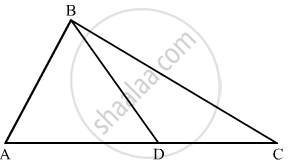Exercise 17.4 | Q 13 | Page 25

If the length of a median of an equilateral triangle is x cm, then its area is

• x2

• $\frac{\sqrt{3}}{2} x^2$

• $\frac{x^2}{\sqrt{3}}$

• $\frac{x^2}{2}$

Exercise 17.4 | Q 14 | Page 25

If every side of a triangle is doubled, then increase in the area of the triangle is

• $100\sqrt{2}$ %

• 200%

•  300%

•  400%

Exercise 17.4 | Q 15 | Page 25

A square and an equilateral triangle have equal perimeters. If the diagonal of the square is $12\sqrt{2}$  cm, then area of the triangle is

• $24\sqrt{2} c m^2$

• $24\sqrt{3} c m^2$

• $48\sqrt{3} c m^2$

• $64\sqrt{3} c m^2$

## Chapter 17: Heron’s Formula

Exercise 17.1Exercise 17.2Exercise 17.3Exercise 17.4## RD Sharma solutions for Mathematics for Class 9 chapter 17 - Heron’s Formula

RD Sharma solutions for Mathematics for Class 9 chapter 17 (Heron’s Formula) include all questions with solution and detail explanation. This will clear students doubts about any question and improve application skills while preparing for board exams. The detailed, step-by-step solutions will help you understand the concepts better and clear your confusions, if any. Shaalaa.com has the CBSE Mathematics for Class 9 solutions in a manner that help students grasp basic concepts better and faster.

Further, we at Shaalaa.com provide such solutions so that students can prepare for written exams. RD Sharma textbook solutions can be a core help for self-study and acts as a perfect self-help guidance for students.

Concepts covered in Mathematics for Class 9 chapter 17 Heron’s Formula are Area of a Triangle by Heron's Formula, Application of Heron’s Formula in Finding Areas of Quadrilaterals, Area of a Triangle.

Using RD Sharma Class 9 solutions Heron’s Formula exercise by students are an easy way to prepare for the exams, as they involve solutions arranged chapter-wise also page wise. The questions involved in RD Sharma Solutions are important questions that can be asked in the final exam. Maximum students of CBSE Class 9 prefer RD Sharma Textbook Solutions to score more in exam.

Get the free view of chapter 17 Heron’s Formula Class 9 extra questions for Mathematics for Class 9 and can use Shaalaa.com to keep it handy for your exam preparation